• end end Here is the output after Mark's suggestion 解决方案 A while back I went through the code of the imresize function in the MATLAB Image Processing Toolbox to create a simplified version for ...I am trying to write my own function for scaling up an input image by using the Nearest-neighbor interpolation algorithm. The bad part is I am able to see how it works but cannot find the algorithm itself. I will be grateful for any help.
Here's what I tried for scaling up the input image by a factor of 2:
function output = nearest(input)
[x,y]=size(input);
output = repmat(uint8(0),x*2,y*2);
[newwidth,newheight]=size(output);
for i=1:y
for j=1:x
xloc = round ((j * (newwidth+1)) / (x+1));
yloc = round ((i * (newheight+1)) / (y+1));
output(xloc,yloc) = input(j,i);
end
end
Here is the output after Mark's suggestion解决方案
A while back I went through the code of the imresize function in the MATLAB Image Processing Toolbox to create a simplified version for just nearest neighbor interpolation of images. Here's how it would be applied to your problem:
%# Initializations:
scale = [2 2]; %# The resolution scale factors: [rows columns]
oldSize = size(inputImage); %# Get the size of your image
newSize = max(floor(scale.*oldSize(1:2)),1); %# Compute the new image size
%# Compute an upsampled set of indices:
rowIndex = min(round(((1:newSize(1))-0.5)./scale(1)+0.5),oldSize(1));
colIndex = min(round(((1:newSize(2))-0.5)./scale(2)+0.5),oldSize(2));
%# Index old image to get new image:
outputImage = inputImage(rowIndex,colIndex,:);
Another option would be to use the built-in interp2 function, although you mentioned not wanting to use built-in functions in one of your comments.
EDIT: EXPLANATION
In case anyone is interested, I thought I'd explain how the solution above works...
newSize = max(floor(scale.*oldSize(1:2)),1);
First, to get the new row and column sizes the old row and column sizes are multiplied by the scale factor. This result is rounded down to the nearest integer with floor. If the scale factor is less than 1 you could end up with a weird case of one of the size values being 0, which is why the call to max is there to replace anything less than 1 with 1.
rowIndex = min(round(((1:newSize(1))-0.5)./scale(1)+0.5),oldSize(1));
colIndex = min(round(((1:newSize(2))-0.5)./scale(2)+0.5),oldSize(2));
Next, a new set of indices is computed for both the rows and columns. First, a set of indices for the upsampled image is computed: 1:newSize(...). Each image pixel is considered as having a given width, such that pixel 1 spans from 0 to 1, pixel 2 spans from 1 to 2, etc.. The "coordinate" of the pixel is thus treated as the center, which is why 0.5 is subtracted from the indices. These coordinates are then divided by the scale factor to give a set of pixel-center coordinates for the original image, which then have 0.5 added to them and are rounded off to get a set of integer indices for the original image. The call to min ensures that none of these indices are larger than the original image size oldSize(...).
outputImage = inputImage(rowIndex,colIndex,:);
Finally, the new upsampled image is created by simply indexing into the original image.

展开全文• function nearest_neighbor ...%最近邻插值 %输入图像文件及放大倍数 %输出根据放大倍数变化后的新图像 ima=imread(filename); [row,col,color]=size(ima); row_n=round(row*N); col_n=round(col*N); ima_new=zero...
function nearest_neighbor = nearest_neighbor( filename,N )
%最近邻插值
%输入图像文件及放大倍数
%输出根据放大倍数变化后的新图像

[row,col,color]=size(ima);
row_n=round(row*N);
col_n=round(col*N);

ima_new=zeros(row_n,col_n,color,class(ima));

for i=1:row_n
for j=1:col_n
for n=1:color
m=round(i/N);
k=round(j/N);
if m<1
m=1;
end
if k<1
k=1;
end
if m>row
m=row;
end
if k>col
k=col;
end
ima_new(i,j,n)=ima(m,k,n);
end
end
end

imwrite(ima_new,'C:\Users\10594\Desktop\new_filename.jpg');

end



展开全文• 编写程序通过最近邻插值和双线性插值等算法将用户所选取的图像区域进行放大和缩小整数倍的操作，并保存，比较几种插值的效果。
• 1. 最邻近插值原理
MATLAB图像插值算法文章集：
插值函数及其原理 https://blog.csdn.net/Effend/article/details/82870144最近邻插值 https://blog.csdn.net/Effend/article/details/82897898双线性插值 https://blog.csdn.net/Effend/article/details/82996871双三次插值 （待完成）https://blog.csdn.net/Effend/article/details/82996899
1. 概要
顾名思义，最近邻插值就是在目标像素点上插入离对应原像素点最近的点的像素值，而不考虑图像可能的颜色变化。因此，该算法方法较为单一，原理也较为简单，计算量也是最少的。 但是，这个方法也有着很明显的缺点，那就是锯齿化高，在颜色变化程度越高以及放大率越大的图像上进行插值处理时，锯齿化现象越严重，这是因为该算法没有考虑颜色变化。 该算法作为要讨论的三种插值算法中的基础，研究其中的计算过程也是很有必要的。
2. 最近邻插值算法
接下来，我们依据原理设计相应算法，使用MATLAB语言编写。
算法步骤： （1）用户输入图像文件、放大率； （2）根据放大率进行图像变换； （3）输出变化后的图像。
算法代码：
% 最近邻插值
% 输入图像文件及放大率
% 输出根据放大率变化后的新图像
function nearest_neighbor = nearest_neighbor(filename,R)

% 初始化，读入图像，图像数据为m*n*color

% 变化后图像
[row,col,color] = size(img);    % 获得图像的行列数及色板数
row = round(row*R);     % 新图像行
col = round(col*R);     % 新图像列

% 新图像初始化
% 使用class获得原图像的数据类型，使得新图像数据类型与原图像保持一致
img_new = zeros(row,col,color,class(img));

% 对新图像的行、列、色板赋值
for i = 1:row
for j = 1:col
for n = 1:color
x = round(i/R);
y = round(j/R);
% 为了避免x和y等于0而报错，采取+1处理即可
if x == 0
x = x+1;
end
if y == 0
y = y+1;
end
img_new(i,j,n) = img(x,y,n);
end
end
end

% 显示原图像
figure;
imshow(img);
title("Original Image");

% 显示新图像
figure;
imshow(img_new);
title("New Image");

3. 算法分析
在最近邻插值算法中，最重要的是要找出对应的原图像上的像素点，然后将像素点的值赋给新图像。详细分析如下： （1）通过imread函数获得图像的数据，在变量img中包括了图像的宽度、高度以及颜色； （2）用size获得图像的行列数及色板数，并计算得到新图像的行列数； （3）用计算得到的行列数及色板数创建一个新图像，为了保证图像颜色显示与原图像一致，通过class获得原图像类型，并通过zeros函数获得与原图像类型一致的，数值皆为0的新图像矩阵； （4）对新图像的行、列、色板遍历赋值，对新图像的像素赋值中，需要找到与被赋值像素点对应的原图像上的像素点。由于使用的最近邻插值即是直接找到最靠近的像素直接赋值，所以使用round函数对行、列四舍五入，便可找到最近的像素点，再将原图像像素点的颜色赋值给新图像上对应的像素。（此处感谢TRY2333的评论反馈，round后的x和y需要进行时候为0的判断以避免报错的情况。） （5）显示原图像和新图像。
4. 测试环境：
用2幅.jpg文件格式的图像作为示例运行，分别观察放大率<1及>1的情况，文件分别为img.jpg和img1.jpg，具体图像如下： img.jpg 图像大小720*720img1.jpg 图像大小350*3505. 测试效果：
（1）用img.jpg运行函数，设定放大率R=0.8，即缩小原图像。 结果为：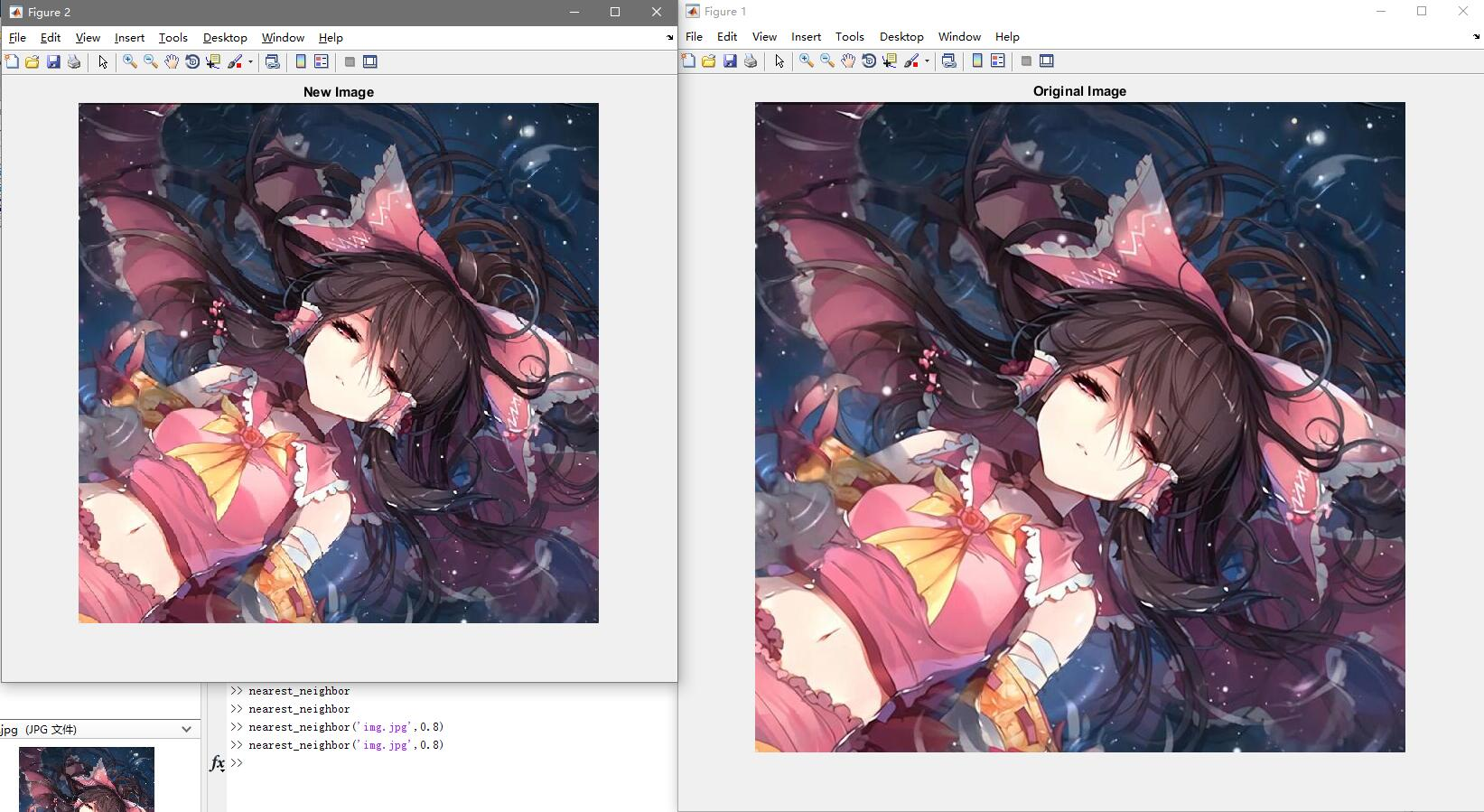可以发现，图像缩小符合预期，色彩也得到保留。 放大图像中的某个部分查看像素点：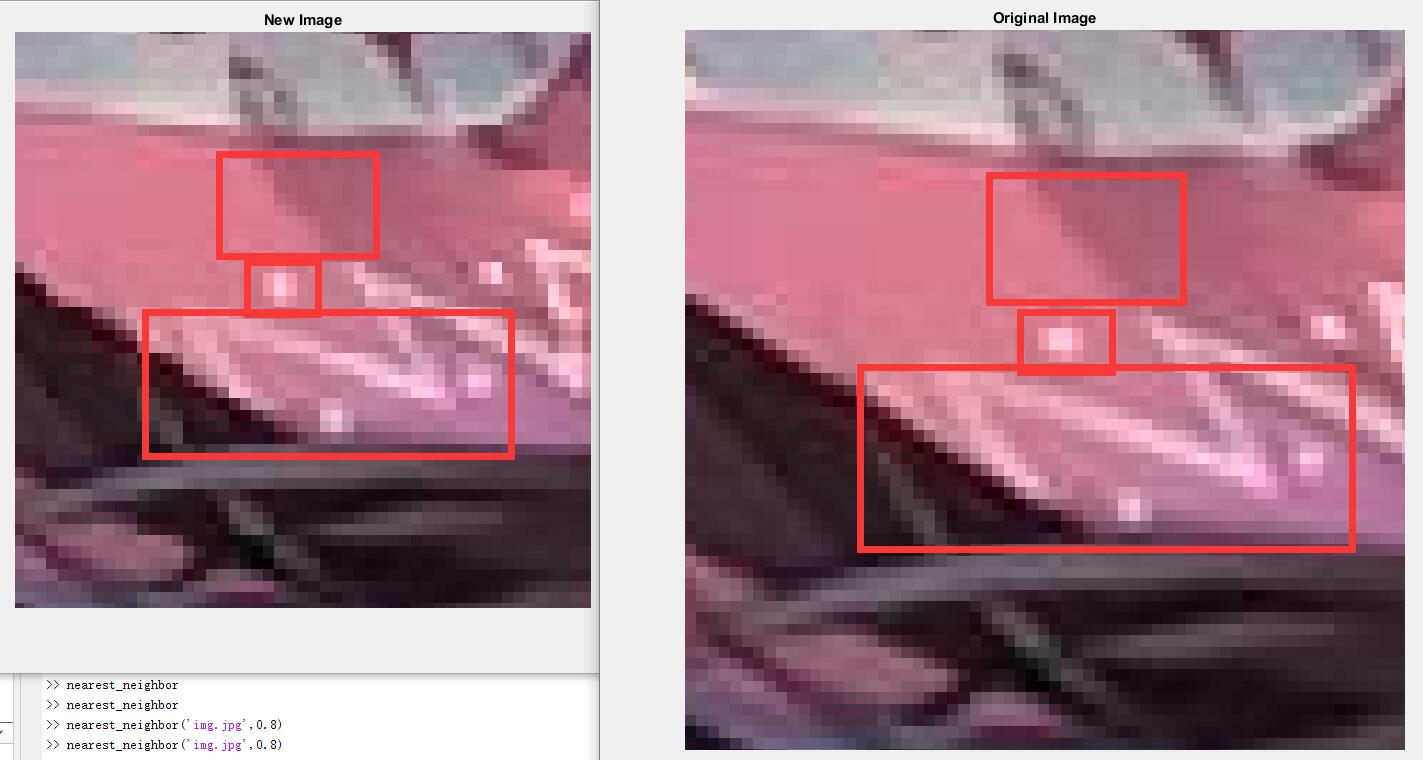从放大后的图像来观察，在用红框圈出的范围内，可以明显发现原图像通过最近邻插值算法缩小后，图像出现了少许的锯齿，并且图像的缩小使得原图像的一些像素丢失，图像精度下降。
（2）用img1.jpg运行函数，设定放大率R=1.5，即放大原图像。 结果为：从上图可以看到，图像放大1.5倍，可以明显观察到锯齿化加深。 放大图像中的某个部分查看像素点：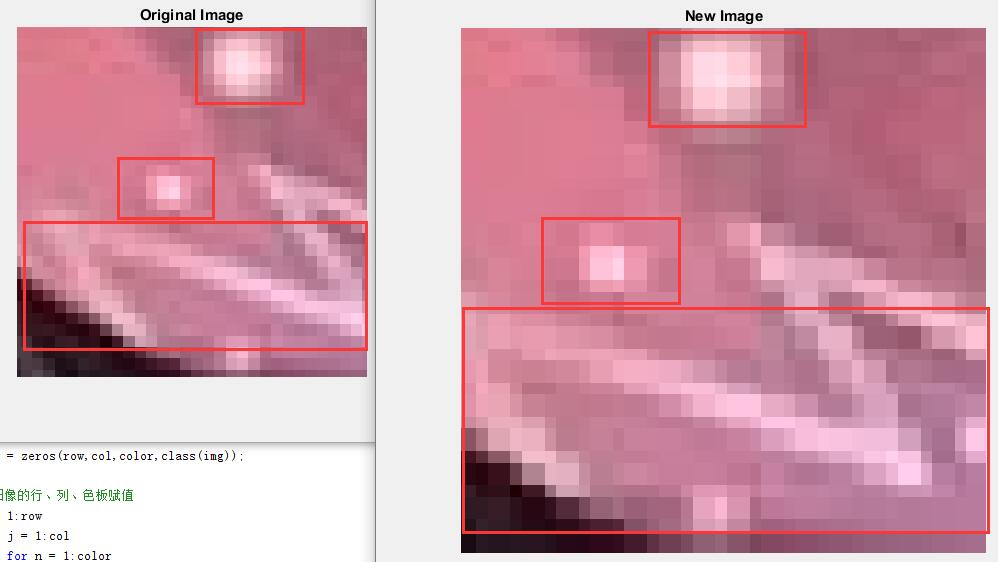在上图中，可以明显观察到新图像中由于放大，需要给新增加的像素点补值，这导致了新图像中出现比原图像更多的像素信息，而由于最近邻插值不会考虑图像颜色的变化，所以图像的锯齿化会加深，而且随着放大率越大，锯齿更为严重。 但在放大率在一定范围内时，图像的放大效果还是很显著的，而且计算速度快。
6. 总结：
最近邻插值的算法可以说是最为暴力的算法，追求简洁高效快速，直接将像素值赋值而抛弃颜色变化的过程。可以看到，无论是缩小图像还是放大图像，都会对图像的细致程度、精度造成影响，图像会呈现更深的锯齿。 但由于其计算速度快，在对于放大或者缩小率要求不高的情况下，使用该算法可以最快完成，而且该算法也无需进行优化操作，使用简单。
–注：本文为原创，未经允许，禁止转载！–
展开全文• 图像插值算法有三种：最近邻插值、双线性插值和双三次插值，这里有前两种插值算法matlab程序。
• 插值算法最近邻插值、双线性插值 插值算法有很多种，这里列出关联比较密切的三种： 最近邻法(Nearest Interpolation)：计算速度最快，但是效果最差。 双线性插值(Bilinear Interpolation)：双线性插值是用原图像...
插值算法：最近邻插值、双线性插值

文章目录
插值算法：最近邻插值、双线性插值最近邻插值法（nearest_neighbor)线性插值单线性插值法双线性插值

插值算法有很多种，这里列出关联比较密切的三种： 最近邻法(Nearest Interpolation)：计算速度最快，但是效果最差。
双线性插值(Bilinear Interpolation)：双线性插值是用原图像中4(2*2)个点计算新图像中1个点，效果略逊于双三次插值，速度比双三次插值快，属于一种平衡美，在很多框架中属于默认算法。
双三次插值(Bicubic interpolation)：双三次插值是用原图像中16(4*4)个点计算新图像中1个点，效果比较好，但是计算代价过大。

最近邻插值法（nearest_neighbor)
1.原理与应用
最近邻插值法nearest_neighbor是最简单的灰度值插值。也称作零阶插值，就是令变换后像素的灰度值等于距它最近的输入像素的灰度值。最近邻插值法可应用于图像的缩放，因为简单的变换与计算，效果一般不好。举例说明其变换过程：
先假设一个2X2像素的图片采用最近邻插值法需要放大到4X4像素的图片，右边？该为多少。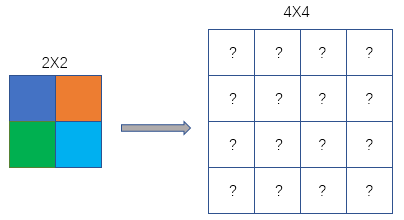2. 公式及计算
最近邻插值法坐标变换计算公式：
srcX=dstX*(srcWidth/dstWidth)
srcY=dstY*(srcHeight/dstHeight)
上式中，dstX与dstY为目标(destination)图像的某个像素的横纵坐标，dstWidth与dstHeight为目标图像的长与宽；srcWidth与srcHeight为原(source)图像的宽度与高度。srcX，srcY为目标图像在该点（dstX，dstY）对应的原图像的坐标。至于公式为啥是这个样，和容易理解，如果是放大图像，(srcWidth/dstWidth)值小于1，同样将dstX同比例映射到原图像的srcX中，如果srcWidth/dstWidth=1，就相当于复制了图片。
图像坐标以左上角为(0,0)坐标。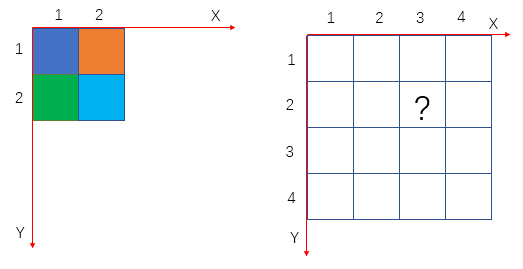右图为经过放大后的目标图像，**？**处的坐标为(3,2)，根据公式计算得到
srcX=3*(2/4)=1.5，srcY=2*(2/4)=1；故？处的像素应该为原图像中的(1.5,1)像素的值，但是像素坐标没有小数，一般采用四舍五入取最邻，所以最终的结果为(2,1)，对应原图像的橙色。其他类比得到放大后的图像：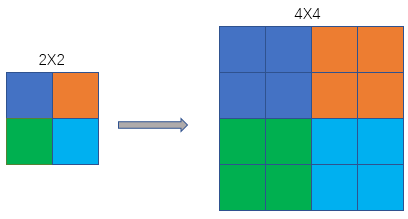线性插值

说双线性之前先说一下单线性，因为在数学上，双线性插值是有两个变量的插值函数的线性插值扩展，其核心思想是在两个方向分别进行一次线性插值。

双线性插值是上采样的一种。在上采样过程中，要恢复图片的大小，提高图片的分辨率，就要用到一些方法，任何可以让图片变成高分辨率的技术都可以称为上采样。

单线性插值法

线性插值法，是指使用连接两个已知量的直线来确定在这两个已知量之间的一个未知量的值的方法。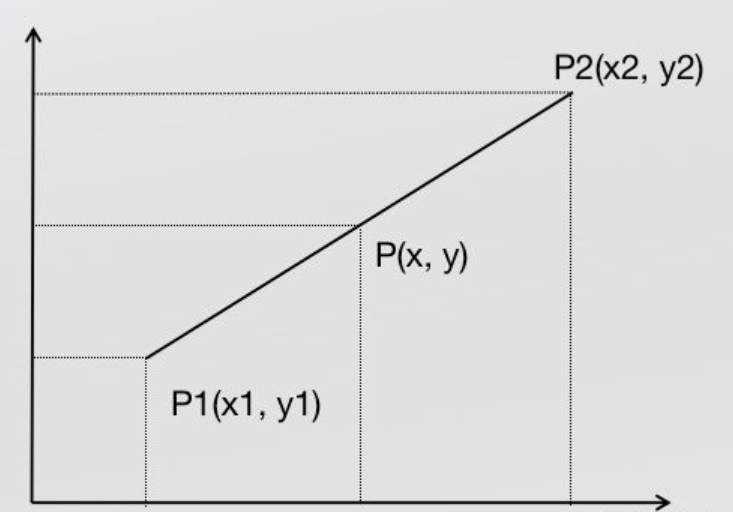根据初中的知识，2点求一条直线公式(这是双线性插值所需要的唯一的基础公式)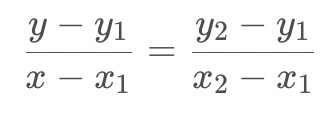经过简单整理成下面的格式：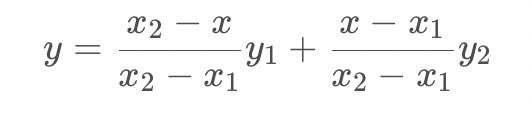这里没有写成经典的AX+B的形式，因为这种形式从权重的角度更好理解。
首先看分子，分子可以看成x与x1和x2的距离作为权重，这也是很好理解的，P点与P1、P2点符合线性变化关系，所以P离P1近就更接近P1，反之则更接近P2。
现在再把公式中的分式看成一个整体，原式可以理解成y1与y2是加权系数，如何理解这个加权，要返回来思考一下，咱们先要明确一下根本的目的：咱们现在不是在求一个公式，而是在图像中根据2个点的像素值求未知点的像素值。这样一个公式是不满足咱们写代码的要求的。
现在根据实际的目的理解，就很好理解这个加权了，y1与y2分别代表原图像中的像素值（x与x1和x2的距离作为权重），上面的公式可以写成如下形式：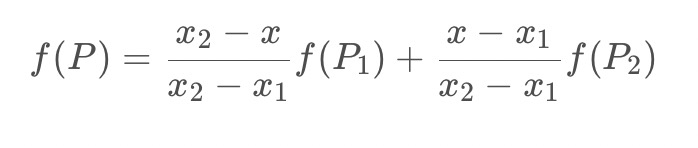双线性插值
根据定义和名字，可以看出就是两个方向的线性插值
假如我们想得到未知函数f在点P= (x,y) 的值，假设我们已知函数f在Q11 = (x1,y1)、Q12 = (x1,y2),Q21 = (x2,y1) 以及Q22 = (x2,y2) 四个点的值。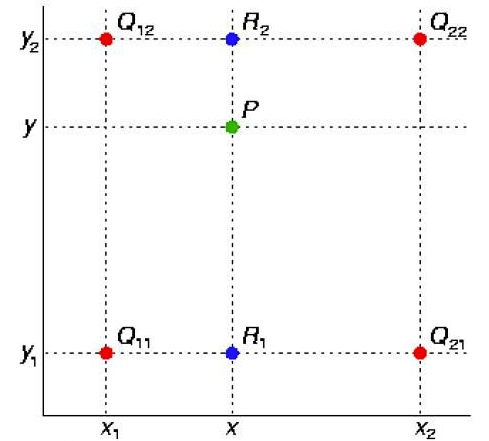首先在x方向进行线性插值，得到R1和R2，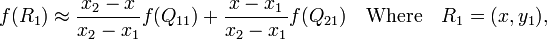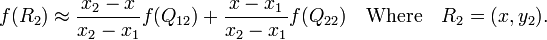然后在y方向进行线性插值，得到P.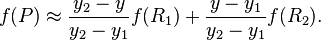整合后的公式如下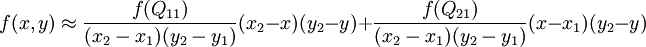2. 图像计算例子
在图像处理的时候，我们先根据
srcX=dstX*(srcWidth/dstWidth)
srcY=dstY*(srcHeight/dstHeight)
来计算目标像素在源图像中的位置（和最近邻插值法一样，但是线性插值的像素是通过公式计算的），这里计算的srcX和srcY一般都是浮点数，
设坐标通过反向变换得到的浮点坐标为 (i+u,j+v) (其中i、j均为浮点坐标的整数部分，u、v为浮点坐标的小数部分，是取值[0,1)区间的浮点数)，则这个像素得值 f(i+u,j+v) 可由原图像中坐标所对应的周围四个像素的值决定，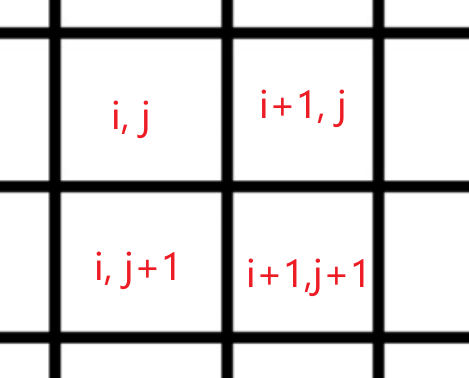计算公式由👆上面整合后的公式替换得到：
f(i+u,j+v) = (1-u)(1-v) f(i,j) + (1-u)v f(i,j+1) + u(1-v) f(i+1,j) + uv f(i+1,j+1)
注：
matlab、openCV对应的resize（）函数的源坐标和目标坐标转换和一般的不一样。
是
SrcX=(dstX+0.5)* (srcWidth/dstWidth) -0.5
SrcY=(dstY+0.5) * (srcHeight/dstHeight)-0.5
python代码
# coding=utf-8
import cv2
import numpy as np
import time

def resize(src, new_size):
dst_w, dst_h = new_size # 目标图像宽高
src_h, src_w = src.shape[:2] # 源图像宽高
if src_h == dst_h and src_w == dst_w:
return src.copy()
scale_x = float(src_w) / dst_w # x缩放比例
scale_y = float(src_h) / dst_h # y缩放比例

# 遍历目标图像，插值
dst = np.zeros((dst_h, dst_w, 3), dtype=np.uint8)
for n in range(3): # 对channel循环
for dst_y in range(dst_h): # 对height循环
for dst_x in range(dst_w): # 对width循环
# 目标在源上的坐标（浮点值）
src_x = (dst_x + 0.5) * scale_x - 0.5
src_y = (dst_y + 0.5) * scale_y - 0.5
# 计算在源图上的（i,j）,和（i+1,j+1）的值
src_x_0 = int(np.floor(src_x))  # floor是下取整
src_y_0 = int(np.floor(src_y))
src_x_1 = min(src_x_0 + 1, src_w - 1)
src_y_1 = min(src_y_0 + 1, src_h - 1)

# 双线性插值
value0 = (src_x_1 - src_x) * src[src_y_0, src_x_0, n] + (src_x - src_x_0) * src[src_y_0, src_x_1, n]
value1 = (src_x_1 - src_x) * src[src_y_1, src_x_0, n] + (src_x - src_x_0) * src[src_y_1, src_x_1, n]
dst[dst_y, dst_x, n] = int((src_y_1 - src_y) * value0 + (src_y - src_y_0) * value1)
return dst

if __name__ == '__main__':
start = time.time()
img_out = cv2.resize(img_in, (600,600))
print('cost %f seconds' % (time.time() - start))

cv2.imshow('src_image', img_in)
cv2.imshow('dst_image', img_out)
cv2.waitKey()

展开全文线性代数 人工智能
• 最近邻插值’nearest’ 什么是最近邻插值？我的理解是它就是用来对新的像素进行赋值的一种方法。 我为了能方便快速的弄清楚它对图像像素的具体操作，我用的是4×4像素的图像，通过imresize()函数来展示。 ...
• 目录一、插值与图像缩放二、最近邻插值1、原理2、代码实现三、双线性插值1、原理2、代码实现 一、插值与图像缩放   首先举个例子说明插值过程，先看看matlab的插值函数 interp() 吧： x = -2 : 1 : 2; y = -2 : 1 ...
• 对图像进行最邻近插值，提高图像的分辨率。 对图像进行最邻近插值，提高图像的分辨率。 对图像进行最邻近插值，提高图像的分辨率。 对图像进行最邻近插值，提高图像的分辨率。
• 2 Matlab实现最近邻插值、双线性插值、双三次插值3最近邻插值的原理简介4双线性插值的原理简介5双三次插值原理简介 1插值是什么？为啥要插值？ 一句话来说就是插值用于图片缩放，为了解决图片缩放中可能出现小数坐标...图片缩放 双线性插值 双三次插值
• MATLAB下利用最近邻算法和双线性插值算法实现灰度图的缩放
• MATLAB实现三种插值算法最近邻内插、双线性内插和双三次内插。包括代码和测试图像结果图像。复现数字图像处理教材内容。
• Kriging插值 最近邻值点插值 距离反比例插值插值
• Bayer数据格式转换为RGB数据格式Modelsim仿真，采用最近邻插值算法，转换效果一般，但实现方式最简单。
• 一、实验目的  1、熟悉并掌握MATLAB工具的使用...2、对图像执行放大、缩小及旋转操作，分别采用最近邻插值、双线性插值及双三次插值方法实现，要求根据算法自己编写代码实现，并分析三种方法的优缺点。 （二）、相关知图像处理
• method为选择插值方法，默认为’nearest’（最近邻插值），还有’bilinear’（双线性插值）、‘bicubic’（双三次插值）。另外还可指定插值内核，此处不作讨论。 3. 三种差值方法原理的简单介绍 （1）最近邻插值...
• 对图像进行旋转，使用最近邻插值法，双线性插值，三次卷积插值三种方法进行插值。源码：clc;clear all;close all;Img=imread('test1.bmp');Img=double(Img);[h w]=size(Img);alpha=pi/6; %逆时针旋转的角度wnew=w*...
• [x0,y0]=meshgrid(-3:0.25:3); z=peaks(x0,y0); [x,y]=meshgrid(-3:0.125:3);...%默认采用了双线性插值算法。 figure title("chushi"); surfl(x0,y0,z); % hold on; figure title("chazhi"); surfl(x,y,z1);
• 最近邻插值（nearest） 算法算法不凭空设定元素点的值，而是选择源图像中最近邻像素点来填充目标图像。 那么，目标元素点所选择的源图像元素点位置应为： srcX=round(dstX/K) srcY=round(dstY/K) 其中，round...
• 当然，最好的情况是你已经用某种语言实现了网上一大堆博客上原创或转载的双线性插值算法，然后发现计算出来的结果和matlab、openCV对应的resize（）函数得到的结果完全不一样。 那这个究竟是怎么回事呢？ ...
• 文章目录最近邻插值（Nearest neighbor interpolation）双线性插值（Bilinear interpolation）双三次插值（Bicubic interpolation） 最近邻插值（Nearest neighbor interpolation） 举例说明： 3X3的深度为8的256级...python 计算机视觉
• 根据上述的算法思想，通过映射点周围的4个点来进行计算，使得新像素点的颜色能具有不同于最近邻插值算法的简单颜色变化。由此使得图像插值算法得到一定改进。 然而，双线性插值算法仍然具有其短板，简单线性变化会...双线性插值 图像算法
• 常见的插值算法有最近邻插值算法，双线性插值算法，三次卷积等最近邻插值算法这是最简单的一种插值算法，根据目标图像（插值后的图像大小）与源图像大小的比值来寻找目标图像的像素位置对应于源图像像素的位置。...图像插值算法 双线性插值法
• clear; close all; clc; CONST=50; A=imread('testImage\hyf1.bmp'); bwimg=im2bw(A); resize_img=[]; [srcWidth srcHeight]=size(bwimg);...% 以下算法思路参考http://blog.csdn.net/ArtX/art...

# matlab最近邻插值算法matlab 订阅